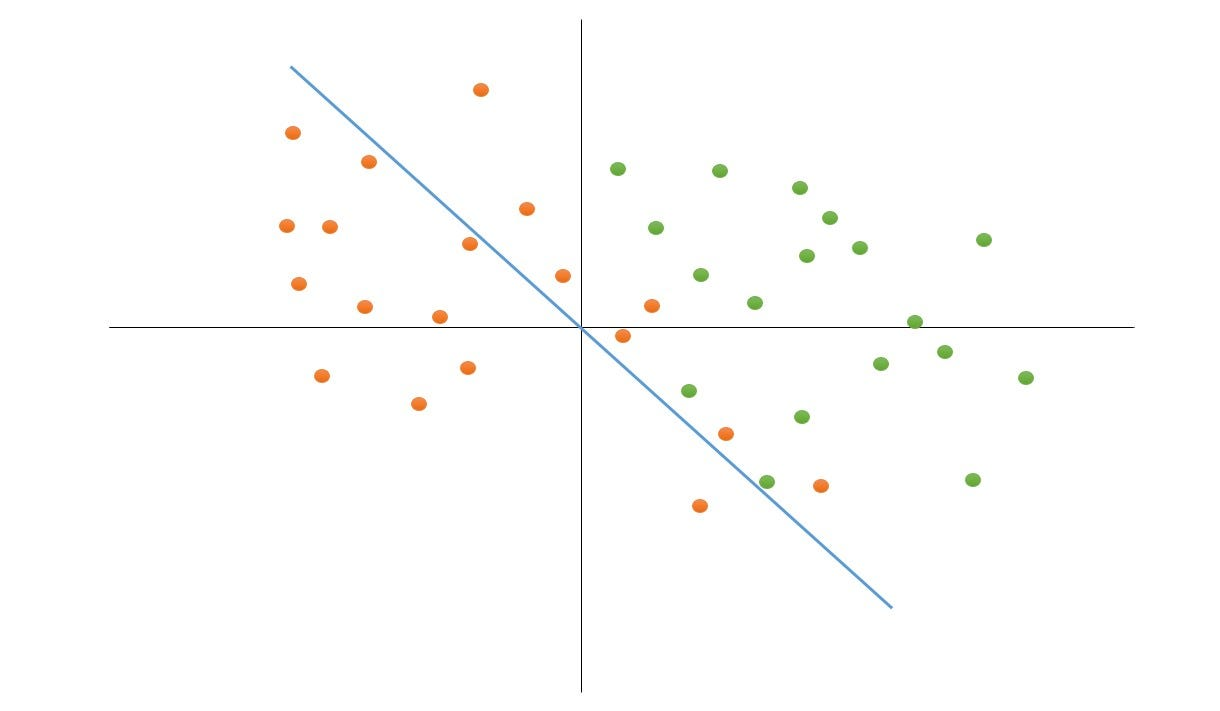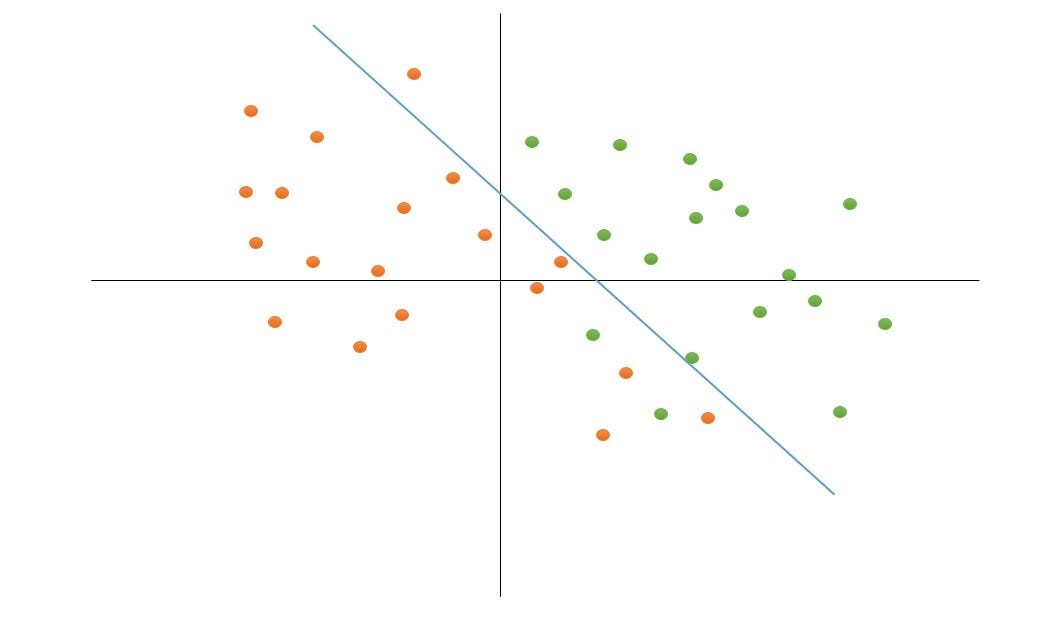# Why and How Bias is used in machine-learning models?

In models such as linear regression and Logistic regression, we have a term named Bias. To get to know why really bias term is used, stay with us and read this article.A scene of desert that the shadow separated it into two parts (src: unsplash.com)

# What is the definition of Bias?

First Of all, let's start with the definition of Bias. Bias means the state of injustice or in other words, It explains that the chance of choosing a group instead of another is higher. If we want to take an example for it in real world, we can say racism is a definition of bias that makes a group more wanted or they are more superior to another group.

Think of a dataset with two groups. The chances of training a model and predicting for both groups is half, But if we give more than half the weight to one group and give it more chance of being chosen in the process of training, The resulting model would have bias and in prediction, It’s more likely to predict the data for the class with higher weight. In this scenario, we gave bias to one of the classes.

# How Bias Distinguish data Mathematically?

The Formula for Linear Regression and Logistic Regression contains a term named bias. It is used to change the baseline of formulas in order to separate the data much better. Here you can see the Linear Regression formula.

As we can see in the image1 there are few terms in this formula. Bias is is number that controls the baseline of the Diagram. The term For weighting each row is called w and error is a random error number. If our data was separated equally in the diagram, The formula with Bias=0 could distinguish data well. But if our data wasn’t separated normally the model needs to have a Bias number of positive or negative in order to learn data better. Let’s look at this problem better with a diagram in the image2.image2. blue angle is the diagram for Linear Regression with Bias=0

In image2 orange and green dots represent data for two groups in the diagram and the blue line is the line of the Linear Regression model with Bias=0. If we look close enough, It’s possible to see that seven orange dots are in the area of green dots. we can move the blue line a bit right and up in order to make the model learn the data better. To achieve that, We can add a number to Bias and move the blue line. In Image3 the Bias number is positive and the Blue line is moved.image3. the blue line is the diagram for Linear Regression with Bias > 0

It’s clear that with Bias>0 in image3 the Linear Regression line is moved and We can see that there are fewer misplaced data in the model. Here We can say that the Linear Regression model has learned the data better.

We Illustrate the need for Bias in Linear Regression. For Logistic Regression everything For the definition of Bias is the same except for the formula, because we’re predicting classes (discrete data) there is a need for another function that wraps the Linear Regression Formula. For Binary Classification (2 values to predict) We need the sigmoid function. We won’t go into detail of sigmoid function because there is plenty of information about it on the internet, But just to know the output of the sigmoid function is between zero and 1 So for binary classification problems, we can assign the data to the first class for the output of zero to half and the other class for more than half.